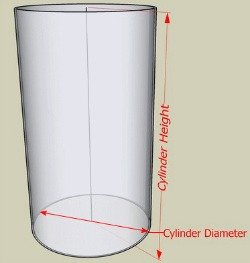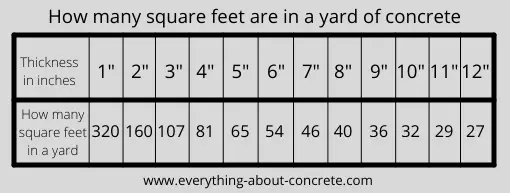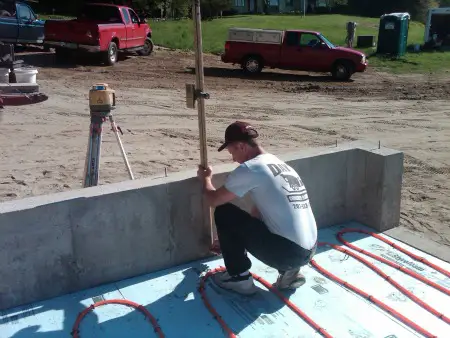# Concrete Cubic Yard Calculator

## the most accurate way of calculating yards of concrete

Use these concrete calculators when you're figuring yards for concrete.

Concrete Calculator

# Concrete Calculator

## Flat Work Concrete Calculator

 Length: Feet Inches Width: Feet Inches Depth: Inches cubic yards of concrete needed

## Wall Concrete Calculator

 Total Length: Feet Inches Height: Feet Inches Thickness: Inches cubic yards of concrete needed

## Cylinder Calculator

 Diameter: Feet Inches Depth: Inches cubic yards of concrete neededUse the above concrete cubic yard calculator for cylinders, sono-tubes, walls, sidewalks and floors.  Just enter in your dimensions, make sure you choose feet and inches carefully, then hit calculate.

An estimated cubic yardage will show in the box. Use these calculators for estimating purposes, there's a formula below to calculate concrete for cylinders if you want to check the calculator.

You can also use my concrete flatwork formula to double check results for slabs and floors.

The information below will help you when figuring yards of concrete for many types of jobs.

## how do i calculate how much concrete i need for a slab?

This is the formula for yards of concrete. (L x W) x H ÷ 27

For a square or rectangular slab:

1. Measure the area in feet to get square footage
2. Multiply length by width, and then by thickness
3. Divide the number by 27 to get cubic yardage.

Example: 20' x 20' slab that's 6" thick

(20 x 20) x .5 = 200

200 ÷ 27 = 7.4 cubic yards of concrete

## how do i calculate square feet into yards of concrete?

To convert your square feet into yards of concrete, you need to know the thickness.

First, you multiply the length by width to get square footage. (keep your measurements in feet)

Second, convert your thickness to feet (6" thick is .5 feet) (4" thick is .33 feet) divide thickness by 12 to convert it to feet.

Third, Multiply your square footage by your thickness to get cubic feet. Then divide by 27 to convert to cubic yards.

Use the diagram below to see how many square feet are in a yard of concrete for each thickness.## how many bags of concrete are in a yard?

If you're using bagged concrete like Sakrete to do your project you'll need to know how many bags to get after you calculate your concrete.

For 1 cubic yard of concrete you'll need:

• 45 bags of 80lb concrete
• 60 bags of 60lb concrete
• 90 bags of 40lb concrete

Check out how I mix concrete by hand here.

## how many yards of concrete in a truck?

Most concrete trucks can carry 10 yards of concrete. The total yardage a truck can carry will depend on if it's a front discharge or rear discharge truck.

Front discharge trucks can carry between 12 - 15 yards of concrete and most rear discharge trucks can carry up to 11 yards of concrete.

## how i calculate yards of concrete

I always check the actual thickness of my floors, patios, decks, or walkways with a laser level.

I check several areas and get an "average" thickness for the entire area. It could be 4", 5", 6" or somewhere in between.

You want to be very accurate here, even being a 1/4" off could mean you don't order enough.

If you don't have a laser you can rent one, it will come in handy when you set the finished grades. Learn how to use a laser level here.

Checking instead of assuming what the actual thickness is will save you from running short on concrete.

Depending on the size of your project, it's always a good idea to add a little extra, 5% to 10%.  When I order concrete, I always order 1/2 to 1 yard extra, over the years I've learned running out of concrete isn't good for me, the customer or the concrete company.That's me checking average thickness of my floor

### how much concrete do i need for a 10' x 10' slab?

A 10' x 10' concrete slab is equal to 100 square feet.

• @ 4" thick you'll need 1.25 yards of concrete
• @ 5" thick you'll need 1.6 yards of concrete
• @ 6" thick you'll need 2 yards of concrete

For each INCH of thickness you'll need approximately .31 yards of concrete for a slab 10' x 10'.

### How to calculate concrete for cylinders and sono-tubes.

Instead of using a concrete calculator to figure your cubic yardage, you can do the math yourself with this formula:

pi X r^2 X h - This will give you the volume in cubic inches which we'll convert to cubic feet then to cubic yards.

pi = 3.14
h = height in inches

Here's an example:

If you have a concrete sono-tube that's 12 inches in diameter and 5 feet deep, here's what the math will look like.

3.14 x (6in. x 6in.) x h
3.14 x 36 x 60 = 6783 cubic inches (rounded up)

To convert the cubic inches to cubic feet divide your result by 1728.  6783/1728 = 3.93 cubic feet.  You can use this measurement to figure out how many bags of concrete to use.

If you divide 3.93 cubic feet by 27 (3.93/27) = .146 cubic yards.  Dividing cubic feet by 27 (a cubic yard) will give you the concrete yardage.  This would be good to know if you had a lot of sono-tubes or a large cylinder to fill.

One more way to share everything-about-concrete.com